Courses

# Capacitors in Series and Parallel Class 12 Notes | EduRev

## Class 12 : Capacitors in Series and Parallel Class 12 Notes | EduRev

The document Capacitors in Series and Parallel Class 12 Notes | EduRev is a part of the Class 12 Course Physics Class 12.
All you need of Class 12 at this link: Class 12

5. Capacitor Circuits

Ex.8 Find charge on each capacitor.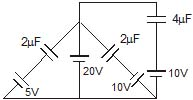Sol. Charge on C1 = C1V1 = 2 × (20 - 5)μC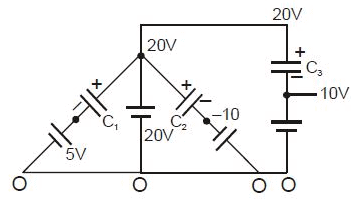= 30 μC

Charge on C2 = C2V2 = 2 × (20 - (-10))μC

= 60 μC

Charge on C3 = C3V3 = 4 × (20 - 10)μC

= 40 μC

Ex.9 Find charge on each capacitor.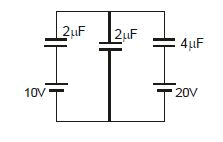Sol. Charge on C1 = (x - 10) C1

Charge on C2 = (x - 0) C2

Charge on C3 = (x - 20) C3

Now from charge conservation at node x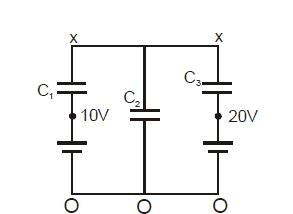(x - 10)C1  (x - 0)C2  (x - 20)C3 = 0

⇒ 2x - 20 2x 4x - 80 = 0

⇒ x = 25 Therefore

so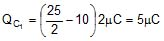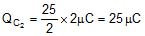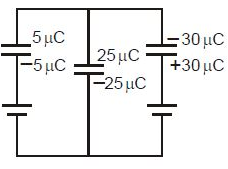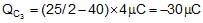Ex.10 In the given circuit find out the charge on each capacitor. (Initially they are uncharged)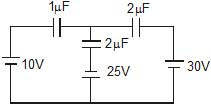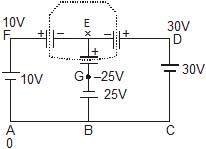Sol. Let potential at A is 0, so at D it is 30 V, at F it is 10 V and at point G potential is -25V. Now apply Kirchhoff's Ist law at point E. (total charge of all the plates connected to 'E' must be same as before i.e. 0)

Therefore, (x - 10) +  (x - 30) 2 +(x 25) 2 = 0

5x = 20

x = 4

Final charges :

Q2mF = (30 - 4) 2 = 52 mC

Q1mF = (10 - 4) = 6 mC

Q2mF = (4 - (-25)) 2 = 58 mC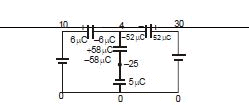Ex.11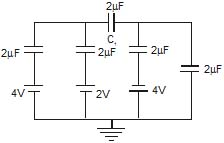Find voltage across capacitor C1.

Sol.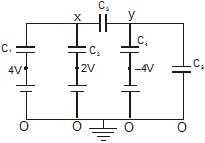Now from charge conservation at node x and y

for x

(x - 4)C1 + (x - 2)C2 + (x - y)C3 = 0 ⇒

2(x - 4) + 2(x - 2) (x - y) 2 = 0

6x - 2y - 12 = 0 .....(1)

For y

(y - x)C3 +  [y -(-4)]C4  (y - 0)C5 = 0 ⇒ (y - x)2 (y 4) 2 y 2 = 0

= 6y - 2x 8 = 0 .....(2)

eq. (1) & (2)

y = - 3 Therefore

x = 7 Therefore

So potential difference = x - y =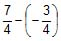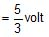6. Combination of Capacitors :

6.1 Series Combination :

(i) When initially uncharged capacitors are connected as shown, then the combination is called series combination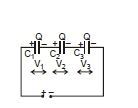(ii) All capacitors will have same charge but different potential difference across then.

(iii) We can say that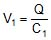V1 = potential across C1

Q = charge on positive plate of C1

C1 = capacitance of capacitor similarly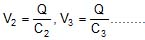(iv) V1 : V2 : V3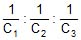We can say that potential difference across capacitor is inversely proportional to its capacitance in series combination.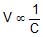Note :  In series combination the smallest capacitor gets maximum potential.

(v)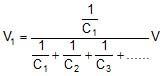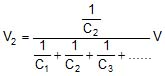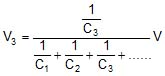Where V = V1 +   V2 + V3

(vi) Equivalent Capacitance :

Equivalent capacitance of any combination is that capacitance which when connected in place of the combination stores same charge and energy as that of the combination

In series :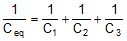........................

In series combination equivalent is always less then smallest capacitor of combination.

(vii) Energy stored in the combination

Ucombination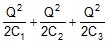Ucombination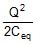Energy supplied by the battery in charging the combination

Ubattery = Q × V = Q .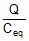=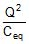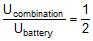Half of the energy supplied by the battery is stored in form of electrostatic energy and half of the energy is converted into heat through resistance.

Derivation of Formulae :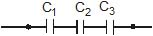Meaning of equivalent capacitor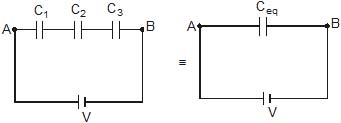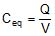Now,

Initially, the capacitor has no charge. Applying Kirchhoff's voltage law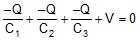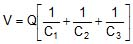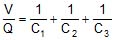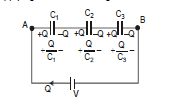in general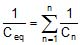Ex.12 Three initially uncharged capacitors are connected in series as shown in circuit with a battery of emf 30V. Find out following :

(i) charge flow through the battery,

(ii) potential energy in 3 mF capacitor.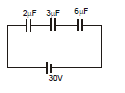(iii) Utotal in capacitors

(iv) heat produced in the circuit

Sol.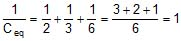Ceq = 1 μF.

(i) Q = Ceq V = 30 μC

(ii) charge on 3μF capacitor = 30 μC

energy =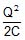=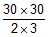= 150 μJ

(iii) Utotal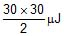= 450 μJ

(iv) Heat produced = (30 μC) (30) - 450 μJ = 450 μJ

Ex.13 Two capacitors of capacitance 1 mF and 2mF are charged to potential difference 20 V and 15 V as shown in figure. If now terminal B and C are connected together terminal A with positive of battery and D with negative terminal of battery then find out final charges on both the capacitor.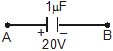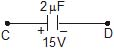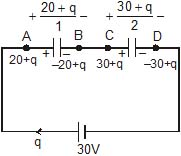Now applying kirchhoff voltage law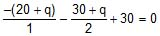- 40 - 2q - 30 - q = - 60

3q = - 10

Charge flow = -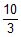μC.

Charge on capacitor of capacitance 1μF = 20 q =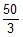Charge on capacitor of capacitance 2μF = 30 q =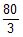6.2 Parallel Combination :

(i) When one plate of one capacitor is connected with one plate of the other capacitor, such combination is called parallel combination.

(ii) All capacitors have same potential difference but different charges.

(iii) We can say that :

Q1 = C1V

Q1 = Charge on capacitor C1

C1 = Capacitance of capacitor C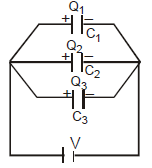V = Potential across capacitor C1

(iv) Q1 : Q2 : Q3 : C1 : C2 : C3

The charge on the capacitor is proportional to its capacitane Q µ C

(v)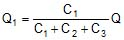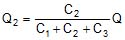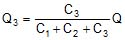Where Q = Q1 + Q2 + Q3 ..............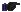• Maximum charge will flow through the capacitor of largest value.

(vi) Equivalent capacitance of parallel combination

Ceq = C1  C2  C3

• Equivalent capacitance is always greater then the largest capacitor of combination.

(vii) Energy stored in the combination :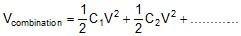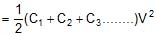=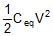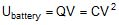• Half of the energy supplied by the battery is stored in the form of electrostatic energy and half of the energy is converted into heat through resistance.

Formulae Derivation for parallel combination :

Q = Q1  Q2  Q3

= C1V C2V C3V

= V(C1  C2  C3)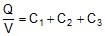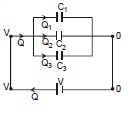Ceq = C1  C2  C3

In general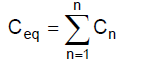Offer running on EduRev: Apply code STAYHOME200 to get INR 200 off on our premium plan EduRev Infinity!

,

,

,

,

,

,

,

,

,

,

,

,

,

,

,

,

,

,

,

,

,

;